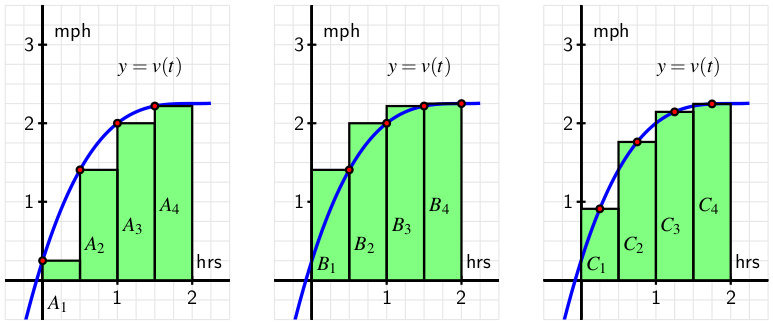A person walking along a straight path has her velocity in miles per hour at time $t$ given by the function $v(t) = 0.25t^3 - 1.5t^2 + 3t + 0.25$, for times in the interval $0 \leq t \leq 2$. The graph of this function is also given in each of the three diagrams below.Note that in each diagram, we use four rectangles to estimate the area under $y = v(t)$ on the interval $[0, 2]$, but the method by which the four rectangles' respective heights are decided varies among the three individual graphs.

Think about how the heights of the rectangles in the left-most diagram are being chosen. Determine the value of $S=A_1+A_2+A_3+A_4$ by evaluating the function $y=v(t)$ at appropriately-chosen values and observing the width of each rectangle. Note, for example, that $A_3 = v(1)\cdot \frac{1}{2} = 2\cdot \frac{1}{2} = 1$.
$S =$

Use the rectangles in the middle diagram to find the value of $T=B_1+B_2+B_3+B_4$.
$T =$

Use the rectangles in the right-most diagram to find the value of $U=C_1+C_2+C_3+C_4$.
$U =$

Which estimate do you think is the best approximation of $D$, the total distance the person traveled on $[0,2]$? Why?

In order to get credit for this problem all answers must be correct.